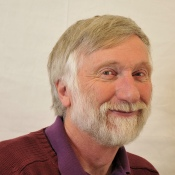# David Aldous# David Aldous

Professor Emeritus
Status
Current
Email
aldous@stat.berkeley.edu
Research Expertise and Interests

analysis of algorithms, applied probability, complex networks, entropy, mathematical probability, phylogenetic trees, random networks, spatial networks, popularization of probability

David Aldous is Professor in the Statistics Dept at U.C. Berkeley, since 1979. He received his Ph.D. from Cambridge University in 1977. He is the author of "Probability Approximations via the Poisson Clumping Heuristic" and (with Jim Fill) of a notorious unfinished online work "Reversible Markov Chains and Random Walks on Graphs". His research in mathematical probability has covered weak convergence, exchangeability, Markov chain mixing times, continuum random trees, stochastic coalescence and spatial random networks. A central theme has been the study of large finite random structures, obtaining asymptotic behavior as the size tends to infinity via consideration of some suitable infinite random structure. He has recently become interested in articulating critically what mathematical probability says about the real world.

He is a Fellow of the Royal Society, and a foreign associate of the National Academy of Sciences.

He does research in mathematical probability. A central theme is the study of large finite random structures, obtaining asymptotic behavior as the size tends to infinity via consideration of some suitable infinite random structure. Much current work involves random network models.

Research Areas
Select Publications
• Stopping times and tightness. Annals of Probability 6 (1978) 335-340.
• Random Walks on Finite Groups and Rapidly Mixing Markov Chains. In Seminaire de Probabilites XVII, Lecture Notes in Math. 986 (1983) 243--297.
• Exchangeability and Related Topics. In Ecole d'Ete St Flour 1983. Springer Lecture Notes in Math. 1117 (1985) 1-198.
• The continuum random tree II: an overview. In Stochastic Analysis (ed. M.T. Barlow and N.H. Bingham), 23-70. ; Cambridge University Press, 1991.
• (with Persi Diaconis). Hammersley's Interacting Particle Process and Longest Increasing Subsequences. Probab. Th. Related Fields 103 (1995), 199-213.
• Deterministic and Stochastic Models for Coalescence (Aggregation, Coagulation): A Review of the Mean-Field Theory for Probabilists. Bernoulli 5 (1999) 3-48.
• The zeta(2) Limit in the Random Assignment Problem. Random Structures and Algorithms 18 (2001) 381-418.
• Stochastic Models and Descriptive Statistics for Phylogenetic Trees. from Yule to Today. Statistical Science 16 (2001) 23-34.
• (with J. Michael Steele) The Objective Method: Probabilistic Combinatorial Optimization and Local Weak Convergence. In Probability on Discrete Structures (Volume 110 of Encyclopaedia of Mathematical Sciences), ed. H. Kesten, p. 1-72. Springer, 2003.
• (with Julian Shun) Connected Spatial Networks over Random Points and a Route-Length Statistic. Statistical Science 25 (2010) 275 - 288.
• Scale-Invariant Random Spatial Networks. Preprint, 2013.